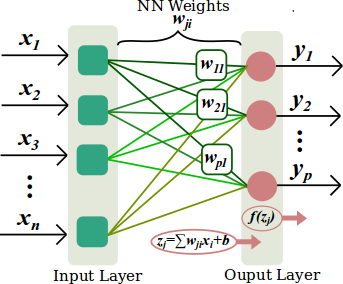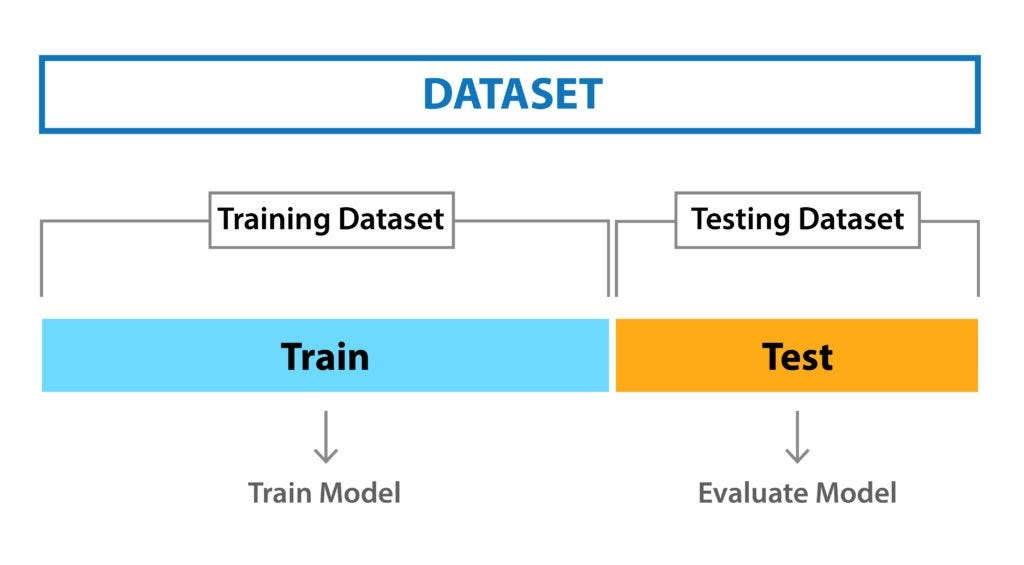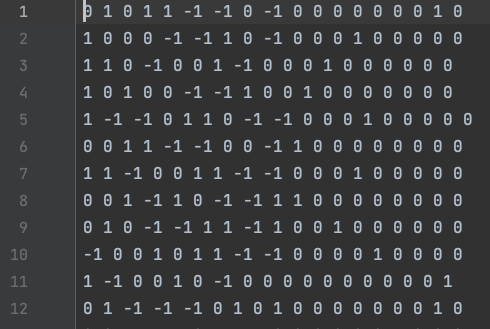# Neural Networks: start with basics and stay for moreFig. 1 — An example of a simple direct propagation network(Source — Gabor Melli’s Research Knowledge Base)
`hidden_1_layer = {‘f_fum’:n_nodes_hl1,'weight':tf.Variable(tf.random_normal([cols_of_model, n_nodes_hl1])),'bias':tf.Variable(tf.random_normal([n_nodes_hl1]))}hidden_2_layer = {'f_fum':n_nodes_hl2,'weight':tf.Variable(tf.random_normal([n_nodes_hl1, n_nodes_hl2])),'bias':tf.Variable(tf.random_normal([n_nodes_hl2]))}hidden_3_layer = {'f_fum':n_nodes_hl3,'weight':tf.Variable(tf.random_normal([n_nodes_hl2, n_nodes_hl3])),'bias':tf.Variable(tf.random_normal([n_nodes_hl3]))}output_layer = {'f_fum':None,'weight':tf.Variable(tf.random_normal([n_nodes_hl3, n_classes])),'bias':tf.Variable(tf.random_normal([n_classes])),}`
`def neural_network_model(data):l1 = tf.add(tf.matmul(data,hidden_1_layer['weight']), hidden_1_layer['bias'])l1 = tf.nn.relu(l1)l2 = tf.add(tf.matmul(l1,hidden_2_layer['weight']), hidden_2_layer['bias'])l2 = tf.nn.relu(l2)l3 = tf.add(tf.matmul(l2,hidden_3_layer['weight']), hidden_3_layer['bias'])l3 = tf.nn.relu(l3)output = tf.matmul(l3,output_layer['weight']) + output_layer['bias']return output`
`def train_neural_network(x):prediction = neural_network_model(x)cost = tf.reduce_mean( tf.nn.softmax_cross_entropy_with_logits(logits = prediction,labels=y) )optimizer = tf.train.AdamOptimizer(learning_rate=0.004).minimize(cost)saver = tf.train.Saver()with tf.Session() as sess:sess.run(tf.global_variables_initializer())train_x,train_y,test_x,test_y = work()for epoch in range(hm_epochs):c = list(zip(train_x, train_y))random.shuffle(c)train_x, train_y = zip(*c)epoch_loss = 0i=0while i < len(train_x):start = iend = i+batch_sizebatch_x = np.array(train_x[start:end])batch_y = np.array(train_y[start:end])_, c = sess.run([optimizer, cost], feed_dict={x: batch_x,y: batch_y})epoch_loss += ci+=batch_sizeif((epoch+1) % 500 == 0):correct = tf.equal(tf.argmax(prediction, 1), tf.argmax(y, 1))accuracy = tf.reduce_mean(tf.cast(correct, 'float'))acc=accuracy.eval({x:test_x, y:test_y})if epoch_loss == 0: breakcorrect = tf.equal(tf.argmax(prediction, 1), tf.argmax(y, 1))accuracy = tf.reduce_mean(tf.cast(correct, 'float'))acc=accuracy.eval({x:test_x, y:test_y})save_path = saver.save(sess, "./modelNext/modelNext.ckpt")`Fig. 2 — Splitting the dataset into Training and Testing datasets (Source — Analytics Vidhya)Fig. 3 — Part of the generated dataset
`def use_neural_network(data):prediction = neural_network_model(x)saver = tf.train.Saver()with tf.Session() as sess:sess.run(tf.global_variables_initializer())saver.restore(sess,"./modelNext/modelNext.ckpt")for i in data:result = (sess.run(tf.argmax(prediction.eval(feed_dict={x:[i]}),1)))`
`move(event) {const cell = +event.target.id;this.board[cell] = -1;if (this.getWinner()) {this.http.post('http://0.0.0.0/api/tic', this.board, httpOptions).toPromise().then((res: any) => {this.board[res] = 1;this.getWinner();});}}`
`def bestmove(input):global graphwith graph.as_default():data = (sess.run(tf.argmax(prediction.eval(session = sess,feed_dict={x:[input]}),1)))return data@app.route('/api/tic', methods=['POST'])def tic_api():data = request.get_json(force=True)data = np.array(data)data = data.tolist()return jsonify(np.asscalar(bestmove(data)))`